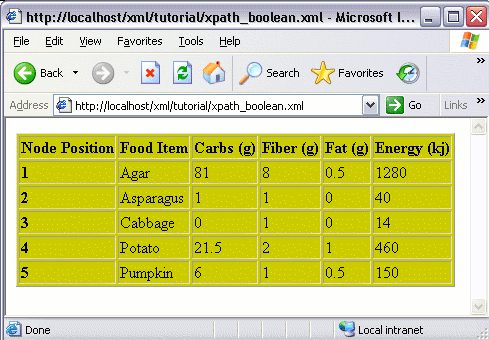# XPath Comparison Operators

XPath expressions can use comparison operators. Comparison operators compare one value to another. The resulting value is a "boolean" value.

A boolean value is one that only returns either true of false. Numbers return false if they are zero, otherwise they return true. Empty strings (i.e. `""`) return false, otherwise they return true.

For example, you could use a comparison operator to check if one number is greater than another number.

## Operators

Here's a list of comparison operators you can use in your XPath expressions:

OperatorDescription
=Is equal to.
!=Is not equal to.
<Is less than.
>Is greater than.
<=Is less than or equal to.
>=Is greater than or equal to.

## Comparison Operator Usage Example

### The Source XML File

Using the same XML file, containing 13 food items and their associated nutritional value:

### The Requirement

This time, instead of displaying all 13 records (as we did in the previous lesson), we only want to display the first 5 records. Like this:### The Solution

We could modify the code from the previous lesson so that only the top 5 records are selected. We could achieve this using the XPath `<=` operator (along with the `position()` function), as follows: